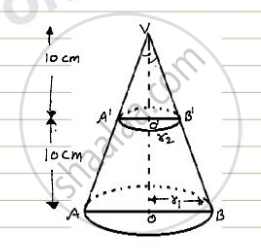Share

# A Metallic Right Circular Cone 20cm High and Whose Vertical Angle is 90° is Cut into Two Parts at the Middle Point of Its Axis by a Plane Parallel to Base. If Frustum So Obtained Bee Drawn into a Wire of Diameter (1/16) Cm Find Length of the Wire? - CBSE Class 10 - Mathematics

ConceptVolume of a Combination of Solids

#### Question

A metallic right circular cone 20cm high and whose vertical angle is 90° is cut into two parts at the middle point of its axis by a plane parallel to base. If frustum so obtained bee
drawn into a wire of diameter
(1/16) cm find length of the wire?

#### Solution

Let VAB be the solid metallic right circular cone of height 20 cm. suppose this cone is cut by a plane parallel to its base of a point O' such that VO' = O'O i.e O' is the mid-point of VO.

Let r1 and r2 be the radii of circular ends of the frustum ABB'A'In triangle VOA we have

tan 45° = (OA)/(VO)

rArr1=r_1/20

rArr r_1 = 20  cm

Now, in Δ VO'A' we have

tan45^@=(O'A')/(VO')

rArr 1= r_2/10

rArr r_2 = 10  cm

∴ volume of the frustum

=1/3pi(r_1^2+r_2^2+r_1r_2)h

Volume of the frustum

(pi)/3(20^2+10^2+20xx10)xx20

=(pi)/3(400+100+200)xx10

=700(pi)/3  cm^3

Let the length of the wire of 1/16 cm

diameter be l cm. then,

radius of wire = 1/32 cm

Volume of the metal used in wire = (pil)/1024 cm^2

Since the frustum is recast into a wire of lenght l cm and diameter 1/16 cm.

∴ Volume of the metal used in wire = Volume of the frustum

rArr (pil)/1024 = 7000(pi)/3

rArr l/1024=7000/3

rArr l= 700/3xx1024

= 2389333.33 cm

= 23893.33 m

Hence, the lenght of wire is 23893.33 m.

Is there an error in this question or solution?
Solution A Metallic Right Circular Cone 20cm High and Whose Vertical Angle is 90° is Cut into Two Parts at the Middle Point of Its Axis by a Plane Parallel to Base. If Frustum So Obtained Bee Drawn into a Wire of Diameter (1/16) Cm Find Length of the Wire? Concept: Volume of a Combination of Solids.
S